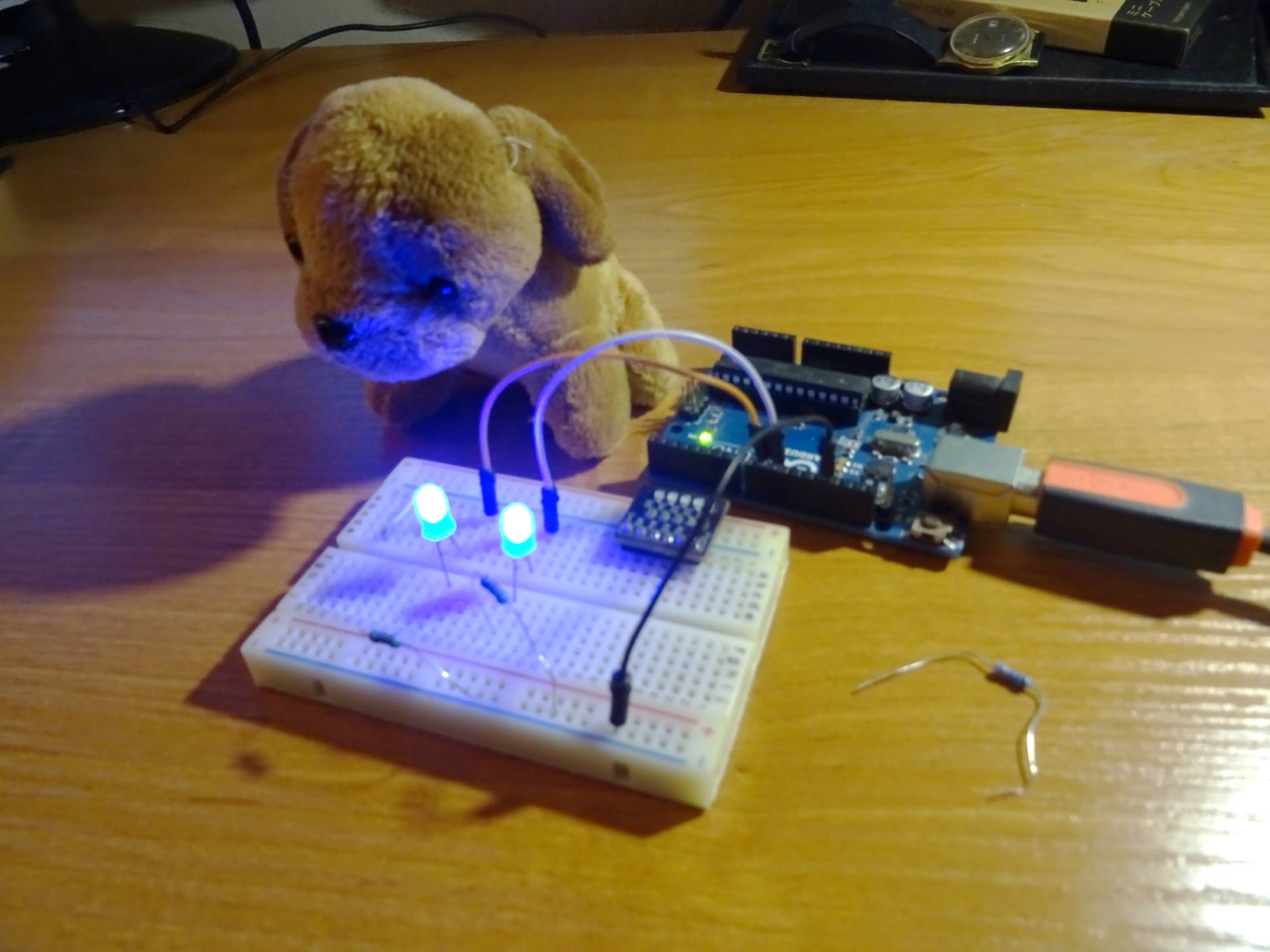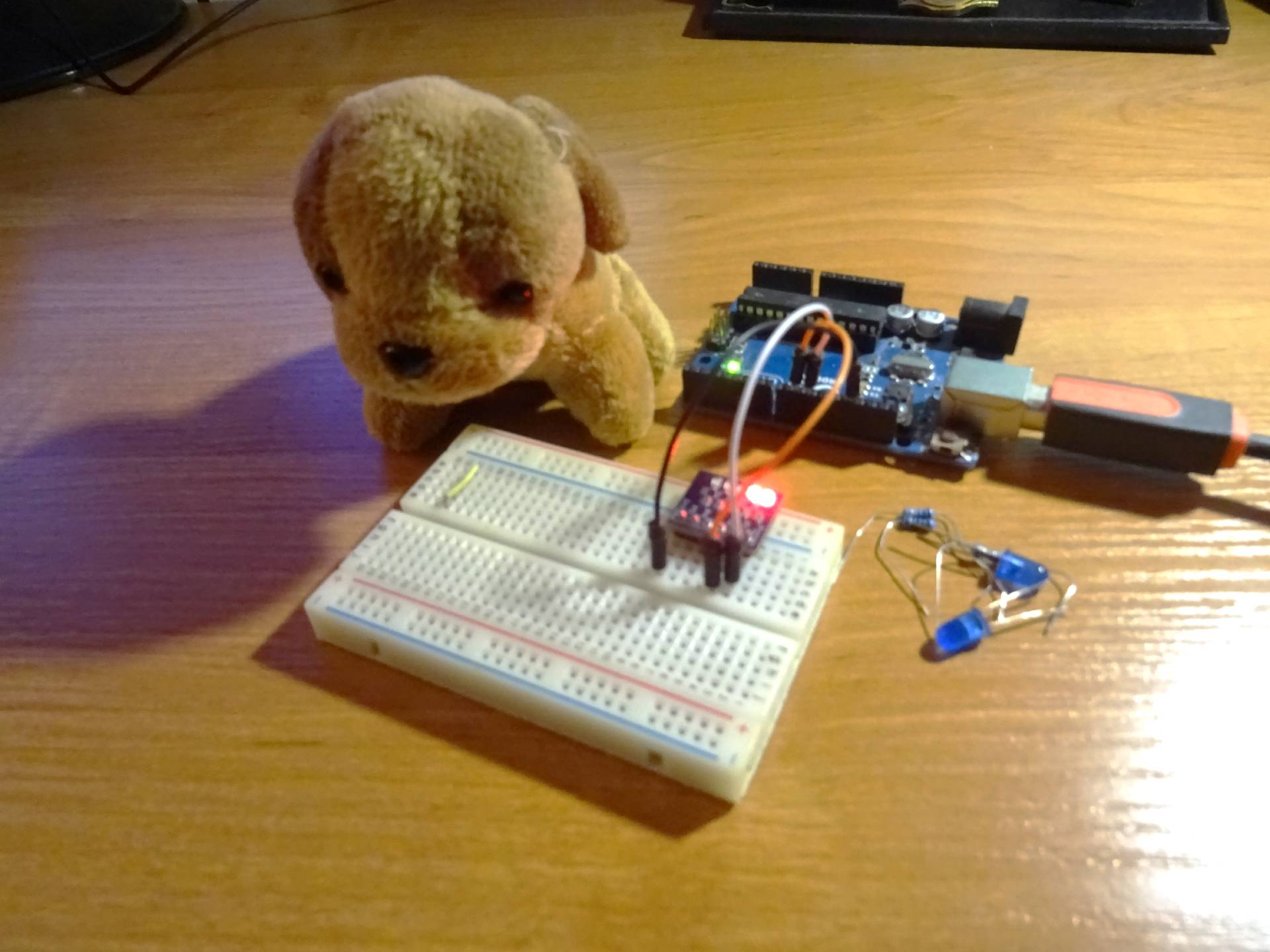# Dog with blue eyes

Zápisník experimentátora

I got a plush dog. The fate of a stuffed animal awaited him, which would end up in a trash can. In this article, we will try to breathe new life into him with the help of Arduino. Or to be more precise, in this article we will just write an algorithm of diabolical blinking of the eyes and only in the next article will we replace them.## Algorithm

Every person knows the devilish flicker of The Hound of the Baskerville. Imagine huge blinking eyes appearing in the dark. They light up or go out at regular intervals. Because we have PWM available, we can improve the effect by gradually turning the LEDs on and off. And now let's try to add a little realism to flickering. If the eyes do not blink completely synchronously, it will seem more believable. Like a living animal that closes one eye a little earlier.## The sketch

The basis of the program is Timer2. It is set to a frequency of 200 Hz. I used the AVR Timer CTC Interrupts Calculator to create the program. The interrupt handler sets the PWM on two LEDs. I used a frequency divider in the program, but if it was set to a higher number, the flicker was too slow, so I finally set the value to one.

The described algorithm is implemented by the class pwmLed. It has three parameters in the constructor. The pin number on which the LED is located. PWM initial value and animation direction. If the PWM value is 255, the direction is reversed and the LED gradually goes out. The same happens if the PWM value is 0. The direction is reversed and the LED lights up gradually. The initial PWM value is the phase shift of the animation. If we want to detune both eyes a bit, we set the value to 0 and 50. This will ensure that the LEDs will be phase shifted by approximately 500 ms.

``````void setupTimer2() {
noInterrupts();
// Clear registers
TCCR2A = 0;
TCCR2B = 0;
TCNT2 = 0;

// 200.32051282051282 Hz (16000000/((77+1)*1024))
OCR2A = 77;
// CTC
TCCR2A |= (1 << WGM21);
// Prescaler 1024
TCCR2B |= (1 << CS22) | (1 << CS21) | (1 << CS20);
// Output Compare Match A Interrupt Enable
TIMSK2 |= (1 << OCIE2A);
interrupts();
}

void setup() {
pinMode(9, OUTPUT);
pinMode(10, OUTPUT);
setupTimer2();
}

class pwmLed {
int led;
int pwm;
int direction;

public:
pwmLed(int _led, int _pwm = 0, int _direction = 1)
: led(_led), pwm(_pwm), direction(_direction)
{}

void Next() {
pwm += direction;
analogWrite(led, pwm);
if (pwm == 255)
direction = -1;
if (pwm == 0)
direction = 1;
}
};

// eyes with phase shift
pwmLed pwm1(9, 0, 1);
pwmLed pwm2(10, 50, 1);

ISR(TIMER2_COMPA_vect) {
static int divider = 0;
if (divider == 0) {
pwm1.Next();
pwm2.Next();
}
divider++;
divider %= 1;
}

void loop() {
}``````

## Source code

The source code of the program is located on the GitHub server.

10.08.2021